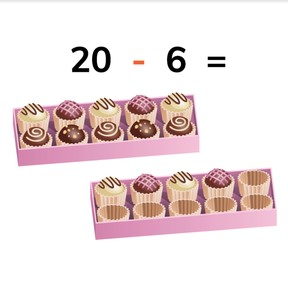Subtraction from 20 with numbers to 20

# Subtraction from 20 with numbers to 208,000 schools use Gynzy92,000 teachers use Gynzy1,600,000 students use Gynzy

## General

Students learn to subtract from 20. They know what the difference is when you subtract a number to 20 from 20.

1.OA.C

## Relevance

Discuss with students that knowing subtraction is useful, so you know how many you have left. If you start with 20 chocolates, and eat 6, you then know that you have 14 chocolates left.

## Introduction

Show the decompositions of 20 on the interactive whiteboard. Drag the correct number and image of beads to the given decompositions.

## Development

Discuss the different subtraction problems that start with 20 on the interactive whiteboard using the example of a farmer and a chicken with 20 eggs. Tell the students that the farmer is coming to take eggs from the chicken. Show the subtraction problems linked to the images. The initial total of 20 eggs does not change, so your subtraction problems always are 20 - however many the farmer takes.
Next show the package of gum on the interactive whiteboard. Show that you start with20 and that you eat 8. Take the 8 away and then count how many you have left.
Students are then asked to determine how many apples are left after a certain amount have been eaten. You start with 20 apples. The apple cores are the eaten apples and have to be taken away.
Remind students that the first number (minuend) is the total amount that you have of the object(s). The second number (subtrahend) tells how many are taken away from the first number. When you take the second number away from the first, you are left with the difference. Students must then fill in the missing numbers. Ask them to write their answers on a sheet of paper and then to hold them up to check their work. You can also choose to have students work with sets of 20 blocks or other manipulatives to determine the difference.
Explain to students that you can also have subtraction problems in which you don't have an image that represents the subtraction problem. You are only given the number 20 and the amount that is taken away. As a class, discuss two subtraction problems and tell students that you start at 20 and count backwards by the amount indicated by the second number. The amount left is the difference. Do a few exercises together and ask students to write the difference on a sheet of paper and to hold it up to check their work. You can also choose to have students work with sets of 20 blocks or other manipulatives to determine the difference.

Check that students are able to subtract from 20 with numbers to 20 by asking the following questions:
- How do you subtract two numbers?
- How do you find the difference in the subtraction problem: 20 - 16 =...?

## Guided practice

Students first are given subtraction problems with visual support. Next they must solve subtraction problems without visual support.

## Closing

Discuss with students that it is important to be able to subtract from 20, so you can calculate subtraction from 20 and determine how much you have left over. To close, give the students a math puzzle. They must solve the subtraction problems to determine which letters fill in the given blanks, and when complete they write a whole sentence.

## Teaching tips

Students who have difficulty with subtraction can make use of MAB blocks or other manipulatives. Have them start by setting out a group of 20 objects and then take different quantities away and solving for the difference.

## Instruction materials

optional: MAB blocks or other manipulatives

### The online teaching platform for interactive whiteboards and displays in schools

• Save time building lessons

• Manage the classroom more efficiently

• Increase student engagement